# Identify Rational and Irrational Numbers

In this worksheet, students will identify if numbers are rational or irrational and use this knowledge to solve problems.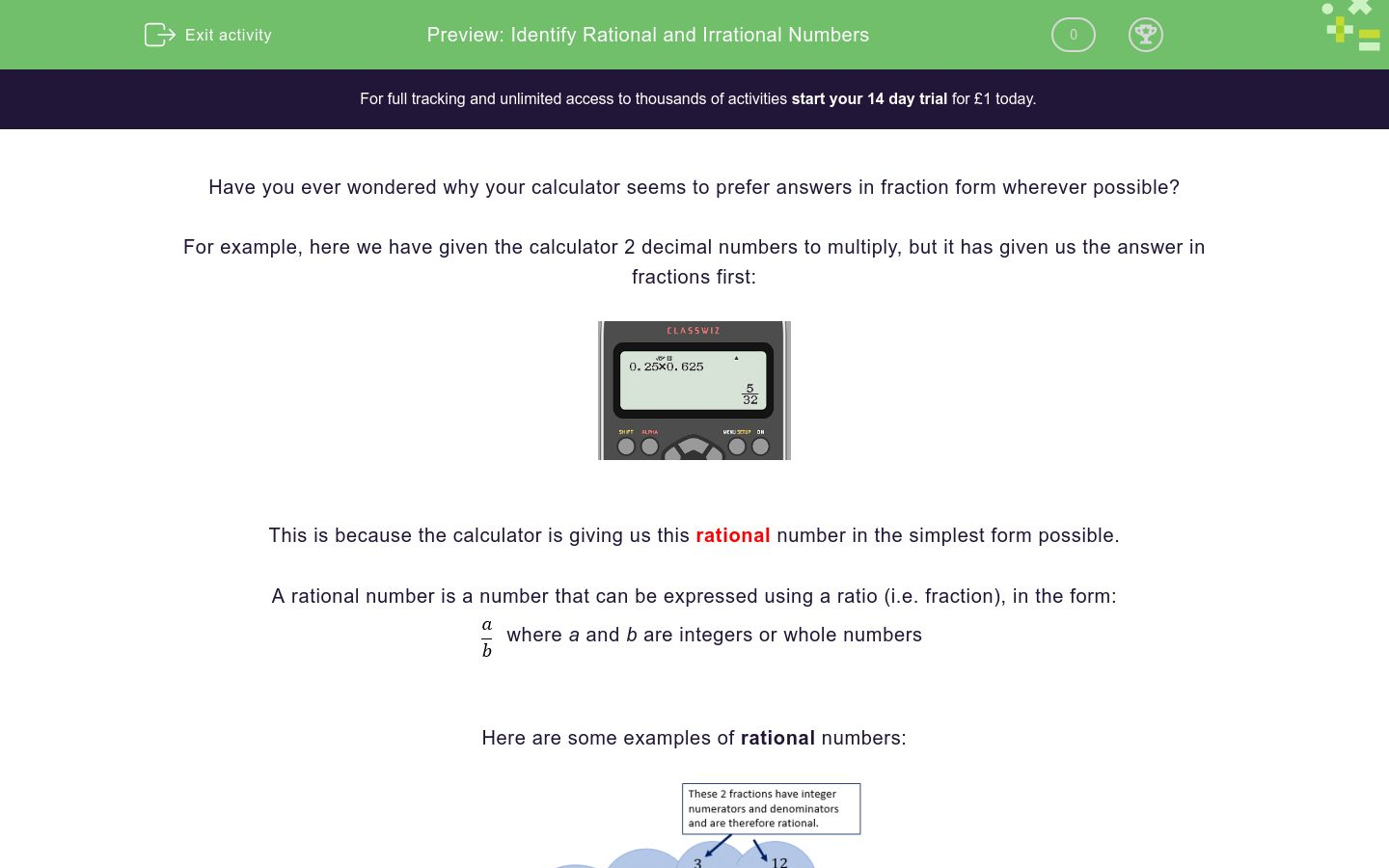Key stage:  KS 4

GCSE Subjects:   Maths

GCSE Boards:   Pearson Edexcel, OCR, Eduqas, AQA

Curriculum topic:   Number, Indices and Surds

Curriculum subtopic:   Structure and Calculation, Exact Calculations

Difficulty level:### QUESTION 1 of 10

Have you ever wondered why your calculator seems to prefer answers in fraction form wherever possible?

For example, here we have given the calculator 2 decimal numbers to multiply, but it has given us the answer in fractions first:This is because the calculator is giving us this rational number in the simplest form possible.

A rational number is a number that can be expressed using a ratio (i.e. fraction), in the form: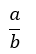where a and b are integers or whole numbers

Here are some examples of rational numbers:However, not all numbers can be written in this form.

Any number that cannot be written in this form is called irrational.

If we ask our calculator to work out a square root, e.g.orThese answers do not terminate and so cannot be written in the form:Remember that a number written under a root sign is called a surd.

These numbers are said to be irrational, as they do not terminate once calculated.

Other examples of irrational numbers include some cube and higher roots, plus numbers expressed as symbols such as π.

In this activity, we will identify if numbers are rational or irrational and use this knowledge to help us solve problems.

Which of the options below show irrational numbers?

8

5/2

4/1

√5

Which of the options in the list below are rational numbers?

√3

√15

√16

∛16

Which of the numbers listed below are rational?

∛16

∛27

∛30

∛(30/2)

Surds are often found when we work with right-angled triangles and Pythagoras' Theorem.

Remember we can find the length of the third side of a right-angled triangle if we know the other 2.Now use this learning to find which one of the triangles below has a hypotenuse with a rational length.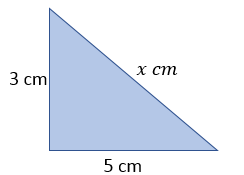Which image from the options gives a rational length for its value of x?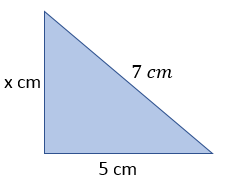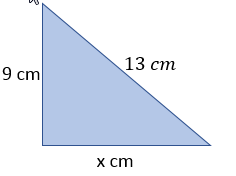Which of the circles below have an irrational value for their circumference?

Remember to use the formula:

C = 2&pi;rWhich of the circles below have an rational value for their area?

Remember to use the formula:

A = πr<sup>2</sup>Which of the values in the list below are rational?

(π)2

(√5)2

(√1.5)2

(√0.4)2

Which triangle from the options will have an irrational value for its area?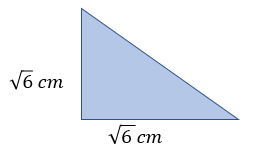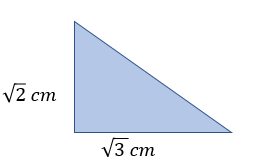Which of the following statements is true about the triangle below?The perimeter of the triangle is rational but the area is irrational

The perimeter of the triangle is irrational but the area is rational

Both the area and the perimeter of the triangle are irrational

Both the area and the perimeter of the triangle are rational

• Question 1

Which of the options below show irrational numbers?

√5
EDDIE SAYS
√5 cannot be written as one integer divided by another, so it is not rational. This is the only irrational number in the list, as can be written as 8/1 and both the other options are already written in the form a/b. Does that make sense? Try calculating the √5 on your calculator to check this is true.
• Question 2

Which of the options in the list below are rational numbers?

√16
EDDIE SAYS
√16 is the only value that can be written in the form a/b. √16 = 4 which we could write as 4/1. All the other surds shown cannot be written in this way and are, therefore, irrational.
• Question 3

Which of the numbers listed below are rational?

∛27
EDDIE SAYS
∛27 = 3 which can be written as 3/1 Did you spot that familiar cube here, as 3 x 3 x 3 = 27? All of the other options cannot be written as one integer divided by another. This may be tricky to spot with: ∛(30/2) If we work out the fraction here, then this sum is ∛15, which is still irrational.
• Question 4

Surds are often found when we work with right-angled triangles and Pythagoras' Theorem.

Remember we can find the length of the third side of a right-angled triangle if we know the other 2.Now use this learning to find which one of the triangles below has a hypotenuse with a rational length.EDDIE SAYS
Option b is the only triangle where working out the value of the hypotenuse gives a number that can be written as one number divided by another: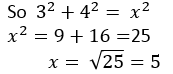• Question 5

Which image from the options gives a rational length for its value of x?EDDIE SAYS
Only triangle c has a side represented by x which is rational. Follow this working through:• Question 6

Which of the circles below have an irrational value for their circumference?

Remember to use the formula:

C = 2&pi;rEDDIE SAYS
As the formula for the circumference of circle involves π, all the circumferences can be expressed most accurately as a multiple of π, which make them all irrational. e.g. Using the first circle, let's find its circumference by substituting 3 for 'r' in this equation. C = 2πr C = 2 x π x 3 = 6π Well done if you spotted this shortcut!
• Question 7

Which of the circles below have an rational value for their area?

Remember to use the formula:

A = πr<sup>2</sup>EDDIE SAYS
To calculate the area of a circle, we need to square the value of the radius and multiply by π. As all the calculations involve π, none of the values involved can be rational. Remember that when symbols are used in maths to represent complex numbers, these are usually going to be irrational.
• Question 8

Which of the values in the list below are rational?

(√5)2
(√1.5)2
(√0.4)2
EDDIE SAYS
This was a bit of a trick question, as a square root (√) and a square (2) cancel each other out. So (√5)2 = 5, which can be expressed as 5/1. Therefore, three of these options are rational. Only (π)2 is irrational.
• Question 9

Which triangle from the options will have an irrational value for its area?EDDIE SAYS
Only option d has an irrational value as its area. Remember that the area of a triangle is found using: A = 1/2 x b x h So if we look at option a and substitute √3 for both b and h, we reach: A = 1/2 x b x h = 1/2 x √3 x √3 Here the square roots cancel each other out, as they equate to a square overall. So A = 1/2 x 9 = 4.5 which can be expressed as a fraction e.g. 45/100. Here is the working for triangle d:• Question 10

Which of the following statements is true about the triangle below?Both the area and the perimeter of the triangle are irrational
EDDIE SAYS
Both the values of this triangle are irrational numbers, therefore, any calculations using them will need to remove the root to create a rational answer. To find the area, we use: A = 1/2 x b x h = 1/2 x √2 x √3 = √3 which is an irrational number To find the perimeter, we need to use Pythagoras' Theorem: a2 + b2 = c2 (√2)2 + (√3)2 = c2 The roots and squares cancel each other out to become: 2 + 3 = c2 So c = √5 which is also an irrational number Therefore, both the area and perimeter of this triangle are irrational values, which the third statement matches. Great work identifying rational and irrational numbers! Why not practise more on circles or triangles so you can put this learning into practise?
---- OR ----

Sign up for a £1 trial so you can track and measure your child's progress on this activity.

### What is EdPlace?

We're your National Curriculum aligned online education content provider helping each child succeed in English, maths and science from year 1 to GCSE. With an EdPlace account you’ll be able to track and measure progress, helping each child achieve their best. We build confidence and attainment by personalising each child’s learning at a level that suits them.

Get started